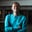Related Tags

laravel
php
communitycreator

# What are Laravel blade control structures?Zubair Idris Aweda

Control structures are code blocks that analyze code and decide which code to run, depending on given parameters.

### Conditionals

#### @if and @endif

@if is used to conditionally render code if the given condition is met. @if is closed by the @endif directive.

#### Example

Suppose you have a variable $age that has a value of 5. @if($test < 5)
Hello World
@endif


#### @else and @elseif

Other times, when the condition in the @if statement evaluates to false, you will want to render some other code. @else lets you render this other code.

@if($test < 5) Hello World @else Hello People @endif  Sometimes, you have more than just a condition. @elseif lets you add more conditions. #### Example @if($test < 5)
Hello World
@elseif($test < 90) Hello 100s @elseif($test < 500)
Hello 500s
@else
Hello People
@endif


#### @unless and @endunless

@unless works as the opposite of @if and only renders code when the condition in it is not met. @unless is closed by @endunless.

Writing @unless($age) works exactly like @if(!$age).

{{ $i }} @endfor  #### @foreach and @endforeach @foreach is used with arrays and ends with endforeach. It gives access to each array item to be used in the view. #### Example @foreach($posts as $post) <h2>{{$post->title }}</h2>
@endforeach


The $loop object has the properties first, last, count, remaining, iteration, and index. #### Example @forelse($posts as $post) <h2>{{$post->title }}</h2>
<p>Loop iteration: {{ $loop->iteration }}</p> <p>Loop index: {{$loop->index }}</p>
<p>Remaining items: {{ $loop->remaining }}</p> <p>Total items count: {{$loop->count }}</p>
<p>Is the first item? {{ $loop->first }}</p> <p>Is the last item? {{$loop->last }}</p>
@empty
No posts yet.
@endforelse


If the loop is in another loop, the $loop object has other properties like depth and parent to tell you how many loops deep you are and reference the parent loop, respectively. #### @forelse, @empy, and @endforelse When using the @foreach loop, sometimes you loop over empty arrays and run into errors. To avoid this, use the @forelse loop. This checks for data in the array first, then loops over the array. If the array is empty, the @empty directive contains code to render. The @forelse loop is closed by the @endforelse directive. #### Example @forelse($posts as $post) <h2>{{$post->title }}</h2>
@empty
No posts yet.
@endforelse


#### @while and @endwhile

The @while directive works exactly like the while loop in native PHP and is ended by the @endwhile directive.

#### Example

@while($item = array_pop($test))
{{ \$item }}
@endwhile


RELATED TAGS

laravel
php
communitycreator

CONTRIBUTORZubair Idris Aweda
RELATED COURSES

View all Courses

Keep Exploring

Learn in-demand tech skills in half the time